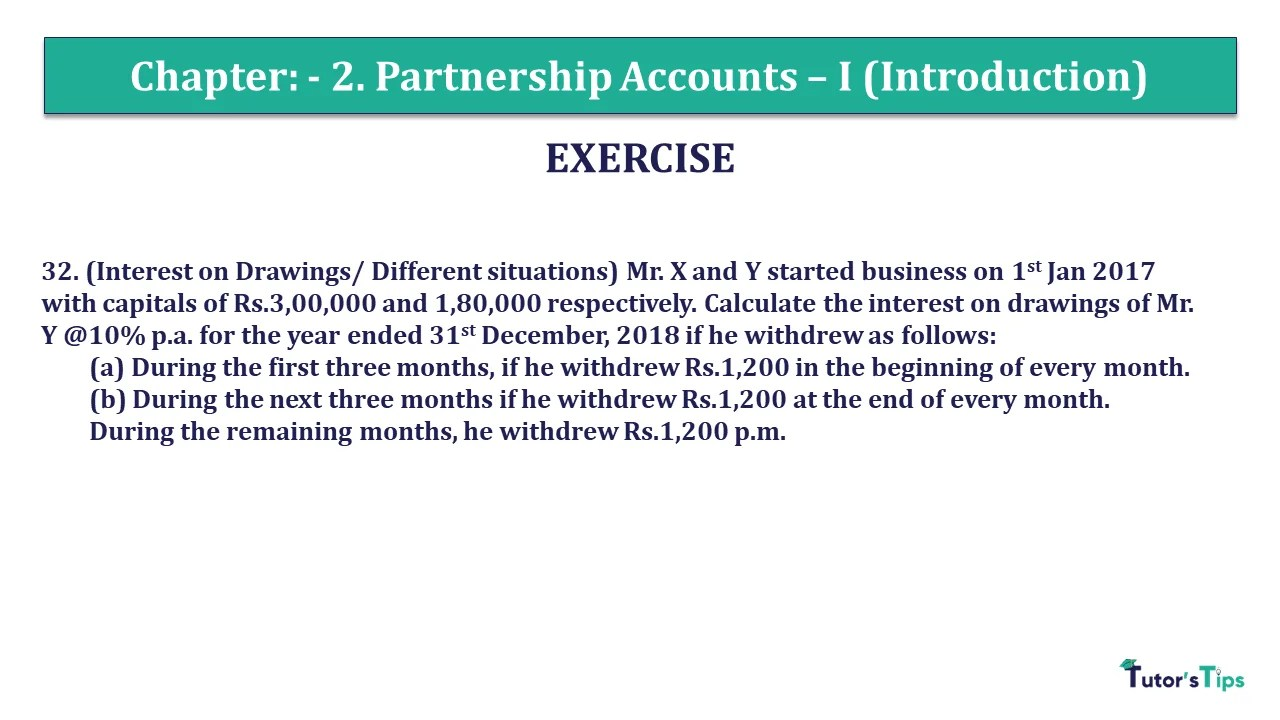# Question 32 Chapter 2 of +2 Part-1 – USHA Publication 12 Class Part – 1Q-32 - CH-2 - Usha +2 Book 2018 - Solution

Question 32 Chapter 2 of +2-Part-1

32. (Interest on Drawings/ Different situations) Mr. X and Y started business on 1st Jan 2017 with capitals of Rs.3,00,000 and 1,80,000 respectively. Calculate the interest on drawings of Mr. Y @10% p.a. for the year ended 31st December, 2018 if he withdrew as follows:
(a) During the first three months, if he withdrew Rs.1,200 in the beginning of every month.
(b) During the next three months if he withdrew Rs.1,200 at the end of every month.
During the remaining months, he withdrew Rs.1,200 p.m.

### The solution of Question 32 Chapter 2 of +2 Part-1: –

Calculation of interest on partner’s Drawings
If partners withdrawal the same amount of drawing on a regular basis then we can calculate the interest on drawing with help of following formula: –
Interest on Drawing = Total Drawings X Rate of Interest X Period/12 Months
Rate of Interest = 10%
Period = the period/time will be calculated with the help of following formula: –

 Period = Time left after 1st withdrawal + Time left after Last withdrawal 2

a) From 1st Jan to 1st March

Y’s withdrawal at the beginning for 3 months = 1,200 p.m. Therefore, total drawings = Rs.1200*3= Rs. 3600.
It means 1st withdrawal has made on 01-01-2017
So, Time left from 1-1-2017 to 31-12-17 in 12 Months
And last withdrawal has made on 1-3-2017
So, Time left from 1-3-2017 to 31-3-2017 in 10 months.

Now, put this time period in the formula

 Period = 12 + 10 2
 Period = 22 2
 Period = 11

Total Drawings = 3,600

 Interest on Drawing = 3,600 X 10 X 11 100 12 Interest on Drawing = 330/-

2. From 30 April to 30 June

Y’s withdrawal at the end of every month = 1,200 p.m. Therefore, total drawings = Rs.1,200*3 = Rs.3600.
It means 1st withdrawal has made on 30-04-2017
So, Time left from 30-4-2017 to 31-12-17 in 8 Months
And last withdrawal has made on 31-6-2017
So, Time left from 30-6-2017 to 31-3-2017 is 6 months.

Now, put this time period in the formula:

 Period = 8 + 6 2
 Period = 14 2
 Period = 7

Total Drawings = 3,600

 Interest on Drawing = 3,600 X 10 X 7 100 12 Interest on Drawing = 210/-

c) Last six months
Y’s withdrawal for last 6 months = 1,200 p.m. Therefore, total drawings = Rs.1200*6= Rs. 7200.
Total Drawings = 7,200

 Interest on Drawing = 7,200 X 10 X 3 100 12 Interest on Drawing = 180/-

Total interest on drawings = Rs. (330+210+180) = Rs.720